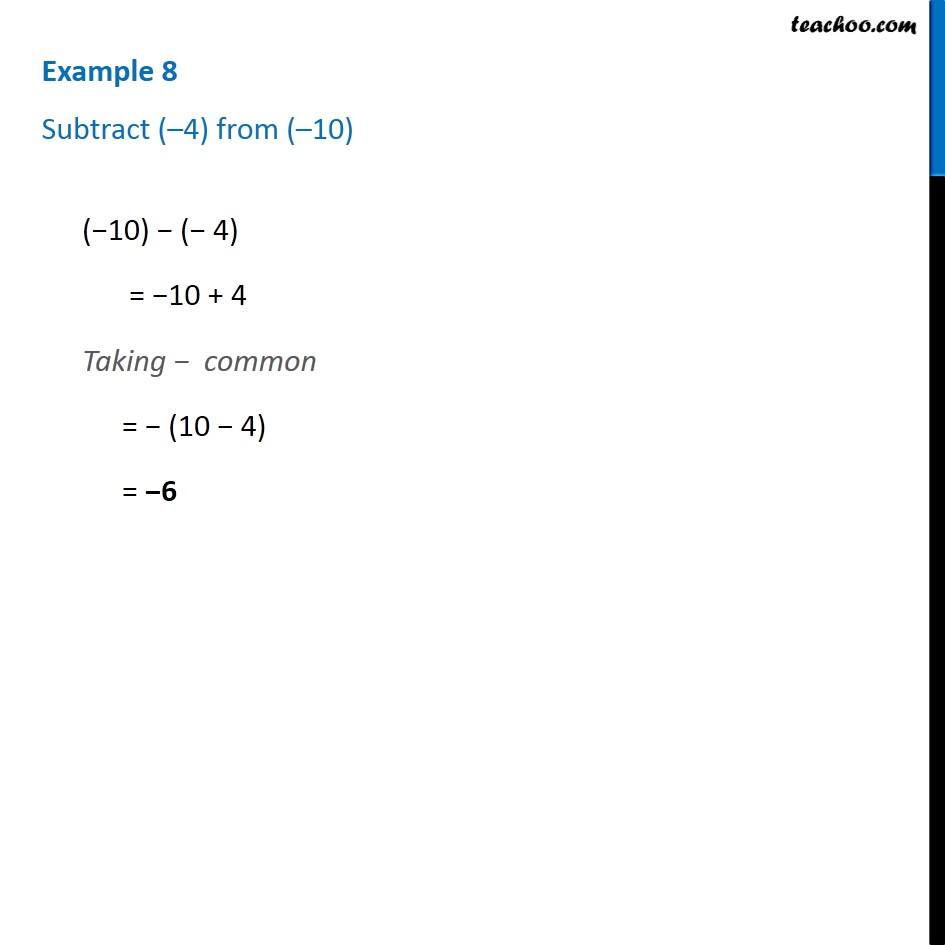1. Chapter 6 Class 6 Integers
2. Serial order wise
3. Examples

Transcript

Example 8 - Chapter 6 Class 6 Integers - NCERT Subtract (–4) from (–10) (−10) − (− 4) = −10 + 4 Taking − common = − (10 − 4) = −6